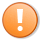# Tutorial 81 | End time before start time?

Determining whether a bar on a chart is between two times is relatively straightforward when the start time is lower than the end time, but what about when the end time is lower than the start time? In other words that start time is today and the end time is tomorrow.Tutorial 81 program applied to a 15 minute @ES chart. Start time is set to 2200 and end time to 0800.

In tutorial 73 I created a simple tutorial to demonstrate how to calculate and draw the highest high and lowest low between two times where the start time was lower than the end time. This tutorial modifies the program created in tutorial 73 so that it will also work if the start time is today and the end time is tomorrow.

This technique should be of use to you as you develop programs that are partially dependent on time of day.

If you are a Gold Pass member you may copy and paste the program below the video.

### Tutorial 81 videoThis content is for members only.